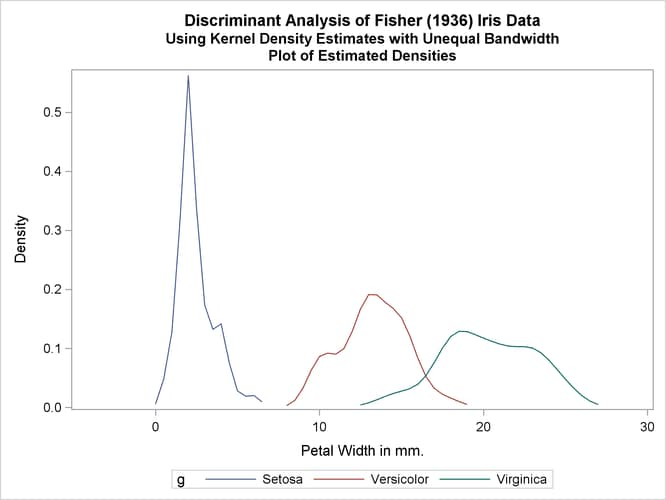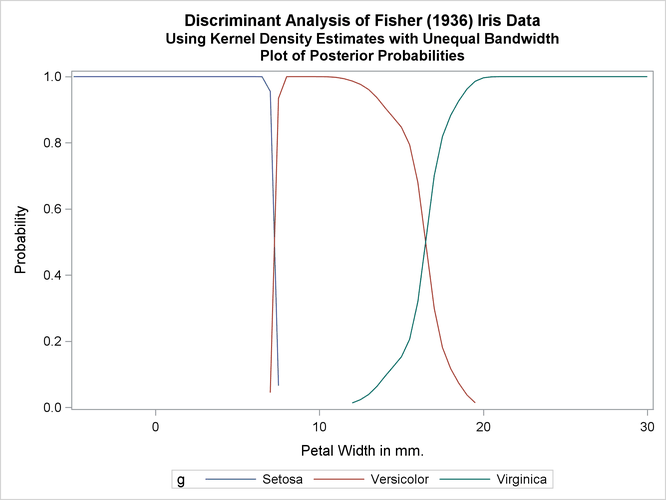# The DISCRIM Procedure

### Example 35.1 Univariate Density Estimates and Posterior Probabilities

In this example, several discriminant analyses are run with a single quantitative variable, petal width, so that density estimates and posterior probabilities can be plotted easily. The example produces Output 35.1.1 through Output 35.1.5. ODS Graphics is used to display the sample distribution of petal width in the three species. For general information about ODS Graphics, see Chapter 21: Statistical Graphics Using ODS. Note the overlap between the species I. versicolor and I. virginica that the bar chart shows. The following statements produce Output 35.1.1:

```title 'Discriminant Analysis of Fisher (1936) Iris Data';

proc freq data=sashelp.iris noprint;
tables petalwidth * species / out=freqout;
run;

proc sgplot data=freqout;
vbar petalwidth / response=count group=species;
keylegend / location=inside position=ne noborder across=1;
run;
```

Output 35.1.1: Sample Distribution of Petal Width in Three Species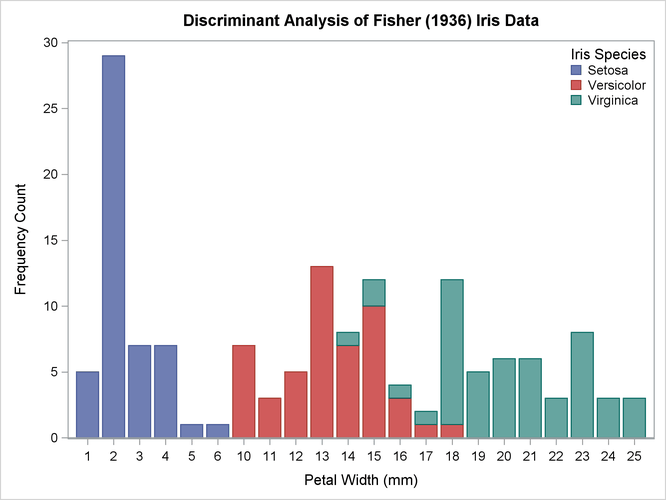In order to plot the density estimates and posterior probabilities, a data set called `plotdata` is created containing equally spaced values from –5 to 30, covering the range of petal width with a little to spare on each end. The `plotdata` data set is used with the TESTDATA= option in PROC DISCRIM. The following statements make the data set:

```data plotdata;
do PetalWidth=-5 to 30 by 0.5;
output;
end;
run;
```

The same plots are produced after each discriminant analysis, so macros are used to reduce the amount of typing required. The macros use two data sets. The data set `plotd`, containing density estimates, is created by the TESTOUTD= option in PROC DISCRIM. The data set `plotp`, containing posterior probabilities, is created by the TESTOUT= option. For each data set, the macros remove uninteresting values (near zero) and create an overlay plot showing all three species in a single plot.

The following statements create the macros:

```%macro plotden;
title3 'Plot of Estimated Densities';

data plotd2;
set plotd;
if setosa     < .002 then setosa     = .;
if versicolor < .002 then versicolor = .;
if virginica  < .002 then virginica  = .;
g = 'Setosa    '; Density = setosa;     output;
g = 'Versicolor'; Density = versicolor; output;
g = 'Virginica '; Density = virginica;  output;
label PetalWidth='Petal Width in mm.';
run;

proc sgplot data=plotd2;
series y=Density x=PetalWidth / group=g;
discretelegend;
run;
%mend;

%macro plotprob;
title3 'Plot of Posterior Probabilities';

data plotp2;
set plotp;
if setosa     < .01 then setosa     = .;
if versicolor < .01 then versicolor = .;
if virginica  < .01 then virginica  = .;
g = 'Setosa    '; Probability = setosa;     output;
g = 'Versicolor'; Probability = versicolor; output;
g = 'Virginica '; Probability = virginica;  output;
label PetalWidth='Petal Width in mm.';
run;

proc sgplot data=plotp2;
series y=Probability x=PetalWidth / group=g;
discretelegend;
run;
%mend;
```

The first analysis uses normal-theory methods (METHOD=NORMAL) assuming equal variances (POOL=YES) in the three classes. The NOCLASSIFY option suppresses the resubstitution classification results of the input data set observations. The CROSSLISTERR option lists the observations that are misclassified under cross validation and displays cross validation error-rate estimates. The following statements produce Output 35.1.2:

```title2 'Using Normal Density Estimates with Equal Variance';

proc discrim data=sashelp.iris method=normal pool=yes
testdata=plotdata testout=plotp testoutd=plotd
short noclassify crosslisterr;
class Species;
var PetalWidth;
run;

%plotden;
%plotprob;
```

Output 35.1.2: Normal Density Estimates with Equal Variance

 Discriminant Analysis of Fisher (1936) Iris Data Using Normal Density Estimates with Equal Variance

The DISCRIM Procedure

 Total Sample Size DF Total 150 149 1 147 3 2

 Number of Observations Read 150 150

Class Level Information
Species Variable
Name
Frequency Weight Proportion Prior
Probability
Setosa Setosa 50 50.0000 0.333333 0.333333
Versicolor Versicolor 50 50.0000 0.333333 0.333333
Virginica Virginica 50 50.0000 0.333333 0.333333

 Discriminant Analysis of Fisher (1936) Iris Data Using Normal Density Estimates with Equal Variance

The DISCRIM Procedure
Classification Results for Calibration Data: SASHELP.IRIS
Cross-validation Results using Linear Discriminant Function

Posterior Probability of Membership in Species
Obs From Species Classified into
Species
Setosa Versicolor Virginica
53 Versicolor Virginica * 0.0000 0.0952 0.9048
100 Versicolor Virginica * 0.0000 0.3828 0.6172
103 Virginica Versicolor * 0.0000 0.9610 0.0390
124 Virginica Versicolor * 0.0000 0.9940 0.0060
130 Virginica Versicolor * 0.0000 0.8009 0.1991
136 Virginica Versicolor * 0.0000 0.9610 0.0390

 * Misclassified observation

 Discriminant Analysis of Fisher (1936) Iris Data Using Normal Density Estimates with Equal Variance

The DISCRIM Procedure
Classification Summary for Calibration Data: SASHELP.IRIS
Cross-validation Summary using Linear Discriminant Function

Number of Observations and Percent Classified
into Species
From Species Setosa Versicolor Virginica Total
Setosa
 50 100
 0 0
 0 0
 50 100
Versicolor
 0 0
 48 96
 2 4
 50 100
Virginica
 0 0
 4 8
 46 92
 50 100
Total
 50 33.33
 52 34.67
 48 32
 150 100
Priors
 0.33333
 0.33333
 0.33333

Error Count Estimates for Species
Setosa Versicolor Virginica Total
Rate 0.0000 0.0400 0.0800 0.0400
Priors 0.3333 0.3333 0.3333

 Discriminant Analysis of Fisher (1936) Iris Data Using Normal Density Estimates with Equal Variance

The DISCRIM Procedure
Classification Summary for Test Data: WORK.PLOTDATA
Classification Summary using Linear Discriminant Function

Observation Profile for Test Data
Number of Observations Read 71
Number of Observations Used 71

Number of Observations and Percent Classified
into Species
Setosa Versicolor Virginica Total
Total
 26 36.62
 18 25.35
 27 38.03
 71 100
Priors
 0.33333
 0.33333
 0.33333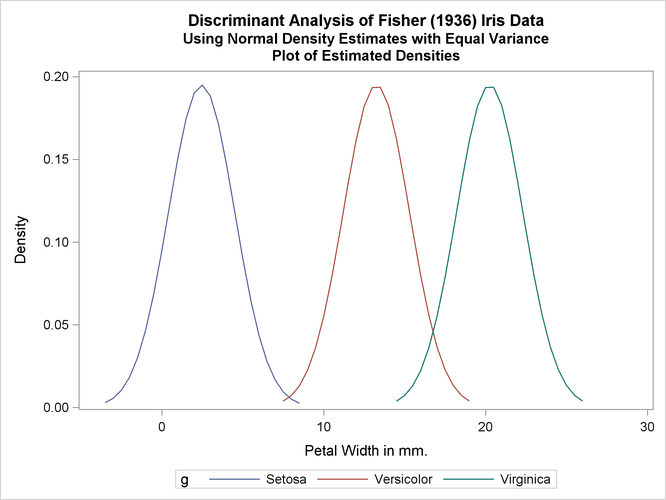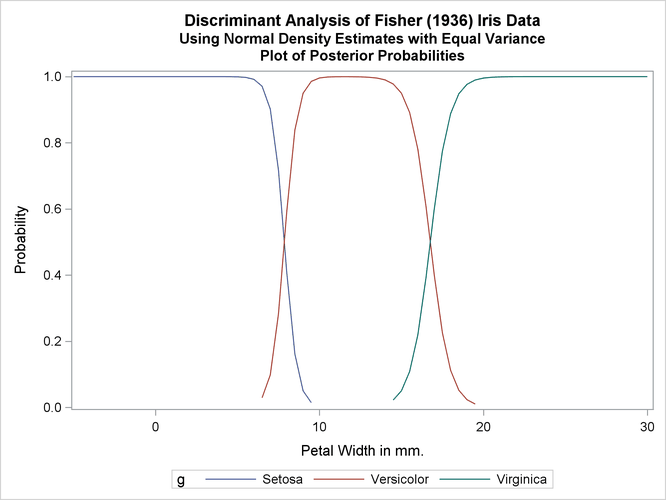The next analysis uses normal-theory methods assuming unequal variances (POOL=NO) in the three classes. The following statements produce Output 35.1.3:

```title2 'Using Normal Density Estimates with Unequal Variance';

proc discrim data=sashelp.iris method=normal pool=no
testdata=plotdata testout=plotp testoutd=plotd
short noclassify crosslisterr;
class Species;
var PetalWidth;
run;

%plotden;
%plotprob;
```

Output 35.1.3: Normal Density Estimates with Unequal Variance

 Discriminant Analysis of Fisher (1936) Iris Data Using Normal Density Estimates with Unequal Variance

The DISCRIM Procedure

 Total Sample Size DF Total 150 149 1 147 3 2

 Number of Observations Read 150 150

Class Level Information
Species Variable
Name
Frequency Weight Proportion Prior
Probability
Setosa Setosa 50 50.0000 0.333333 0.333333
Versicolor Versicolor 50 50.0000 0.333333 0.333333
Virginica Virginica 50 50.0000 0.333333 0.333333

 Discriminant Analysis of Fisher (1936) Iris Data Using Normal Density Estimates with Unequal Variance

The DISCRIM Procedure
Classification Results for Calibration Data: SASHELP.IRIS
Cross-validation Results using Quadratic Discriminant Function

Posterior Probability of Membership in Species
Obs From Species Classified into
Species
Setosa Versicolor Virginica
10 Setosa Versicolor * 0.4923 0.5073 0.0004
53 Versicolor Virginica * 0.0000 0.0686 0.9314
100 Versicolor Virginica * 0.0000 0.2871 0.7129
103 Virginica Versicolor * 0.0000 0.8740 0.1260
124 Virginica Versicolor * 0.0000 0.9602 0.0398
130 Virginica Versicolor * 0.0000 0.6558 0.3442
136 Virginica Versicolor * 0.0000 0.8740 0.1260

 * Misclassified observation

 Discriminant Analysis of Fisher (1936) Iris Data Using Normal Density Estimates with Unequal Variance

The DISCRIM Procedure
Classification Summary for Calibration Data: SASHELP.IRIS
Cross-validation Summary using Quadratic Discriminant Function

Number of Observations and Percent Classified
into Species
From Species Setosa Versicolor Virginica Total
Setosa
 49 98
 1 2
 0 0
 50 100
Versicolor
 0 0
 48 96
 2 4
 50 100
Virginica
 0 0
 4 8
 46 92
 50 100
Total
 49 32.67
 53 35.33
 48 32
 150 100
Priors
 0.33333
 0.33333
 0.33333

Error Count Estimates for Species
Setosa Versicolor Virginica Total
Rate 0.0200 0.0400 0.0800 0.0467
Priors 0.3333 0.3333 0.3333

 Discriminant Analysis of Fisher (1936) Iris Data Using Normal Density Estimates with Unequal Variance

The DISCRIM Procedure
Classification Summary for Test Data: WORK.PLOTDATA
Classification Summary using Quadratic Discriminant Function

Observation Profile for Test Data
Number of Observations Read 71
Number of Observations Used 71

Number of Observations and Percent Classified
into Species
Setosa Versicolor Virginica Total
Total
 23 32.39
 20 28.17
 28 39.44
 71 100
Priors
 0.33333
 0.33333
 0.33333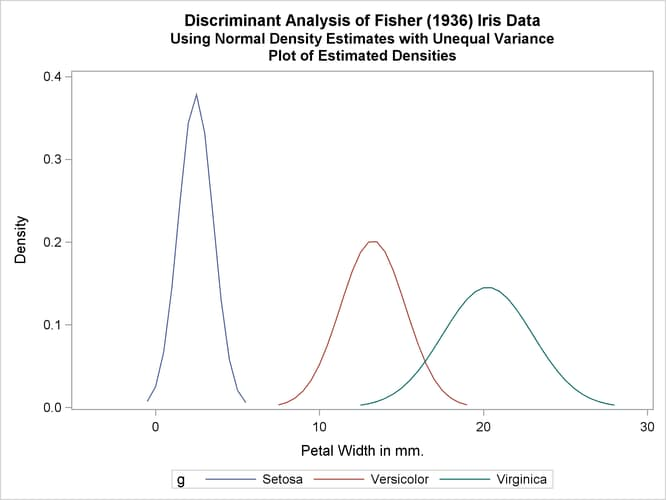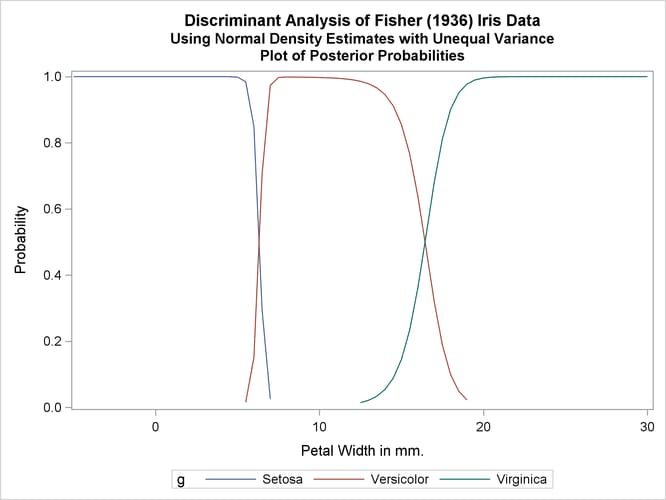Two more analyses are run with nonparametric methods (METHOD=NPAR), specifically kernel density estimates with normal kernels (KERNEL=NORMAL). The first of these uses equal bandwidths (smoothing parameters) (POOL=YES) in each class. The use of equal bandwidths does not constrain the density estimates to be of equal variance. The value of the radius parameter that, assuming normality, minimizes an approximate mean integrated square error is 0.48 (see the section Nonparametric Methods). Choosing r = 0.4 gives a more detailed look at the irregularities in the data. The following statements produce Output 35.1.4:

```title2 'Using Kernel Density Estimates with Equal Bandwidth';

proc discrim data=sashelp.iris method=npar kernel=normal
r=.4 pool=yes
testdata=plotdata testout=plotp
testoutd=plotd
short noclassify crosslisterr;
class Species;
var PetalWidth;
run;

%plotden;
%plotprob;
```

Output 35.1.4: Kernel Density Estimates with Equal Bandwidth

 Discriminant Analysis of Fisher (1936) Iris Data Using Kernel Density Estimates with Equal Bandwidth

The DISCRIM Procedure

 Total Sample Size DF Total 150 149 1 147 3 2

 Number of Observations Read 150 150

Class Level Information
Species Variable
Name
Frequency Weight Proportion Prior
Probability
Setosa Setosa 50 50.0000 0.333333 0.333333
Versicolor Versicolor 50 50.0000 0.333333 0.333333
Virginica Virginica 50 50.0000 0.333333 0.333333

 Discriminant Analysis of Fisher (1936) Iris Data Using Kernel Density Estimates with Equal Bandwidth

The DISCRIM Procedure
Classification Results for Calibration Data: SASHELP.IRIS
Cross-validation Results using Normal Kernel Density

Posterior Probability of Membership in Species
Obs From Species Classified into
Species
Setosa Versicolor Virginica
53 Versicolor Virginica * 0.0000 0.0438 0.9562
100 Versicolor Virginica * 0.0000 0.2586 0.7414
103 Virginica Versicolor * 0.0000 0.8827 0.1173
124 Virginica Versicolor * 0.0000 0.9472 0.0528
130 Virginica Versicolor * 0.0000 0.8061 0.1939
136 Virginica Versicolor * 0.0000 0.8827 0.1173

 * Misclassified observation

 Discriminant Analysis of Fisher (1936) Iris Data Using Kernel Density Estimates with Equal Bandwidth

The DISCRIM Procedure
Classification Summary for Calibration Data: SASHELP.IRIS
Cross-validation Summary using Normal Kernel Density

Number of Observations and Percent Classified
into Species
From Species Setosa Versicolor Virginica Total
Setosa
 50 100
 0 0
 0 0
 50 100
Versicolor
 0 0
 48 96
 2 4
 50 100
Virginica
 0 0
 4 8
 46 92
 50 100
Total
 50 33.33
 52 34.67
 48 32
 150 100
Priors
 0.33333
 0.33333
 0.33333

Error Count Estimates for Species
Setosa Versicolor Virginica Total
Rate 0.0000 0.0400 0.0800 0.0400
Priors 0.3333 0.3333 0.3333

 Discriminant Analysis of Fisher (1936) Iris Data Using Kernel Density Estimates with Equal Bandwidth

The DISCRIM Procedure
Classification Summary for Test Data: WORK.PLOTDATA
Classification Summary using Normal Kernel Density

Observation Profile for Test Data
Number of Observations Read 71
Number of Observations Used 71

Number of Observations and Percent Classified
into Species
Setosa Versicolor Virginica Total
Total
 26 36.62
 18 25.35
 27 38.03
 71 100
Priors
 0.33333
 0.33333
 0.33333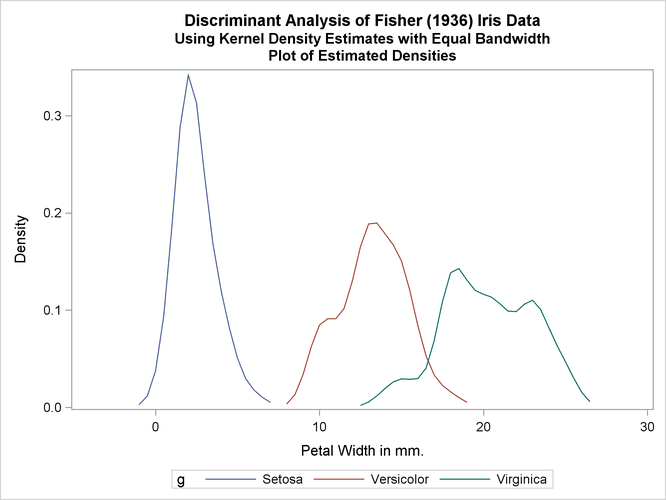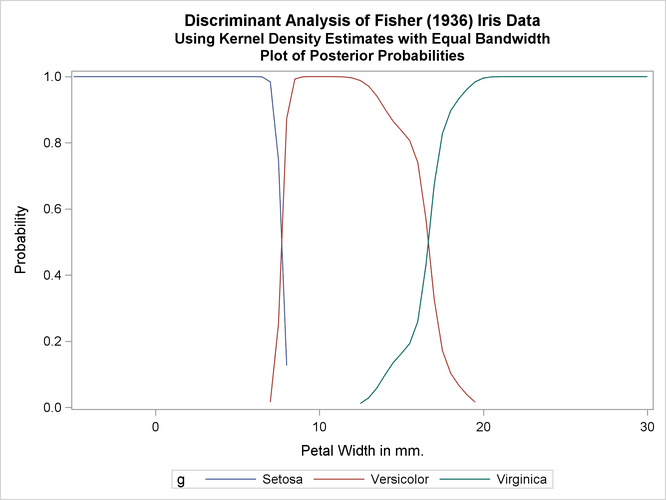Another nonparametric analysis is run with unequal bandwidths (POOL=NO). The following statements produce Output 35.1.5:

```title2 'Using Kernel Density Estimates with Unequal Bandwidth';

proc discrim data=sashelp.iris method=npar kernel=normal
r=.4 pool=no
testdata=plotdata testout=plotp
testoutd=plotd
short noclassify crosslisterr;
class Species;
var PetalWidth;
run;

%plotden;
%plotprob;
```

Output 35.1.5: Kernel Density Estimates with Unequal Bandwidth

 Discriminant Analysis of Fisher (1936) Iris Data Using Kernel Density Estimates with Unequal Bandwidth

The DISCRIM Procedure

 Total Sample Size DF Total 150 149 1 147 3 2

 Number of Observations Read 150 150

Class Level Information
Species Variable
Name
Frequency Weight Proportion Prior
Probability
Setosa Setosa 50 50.0000 0.333333 0.333333
Versicolor Versicolor 50 50.0000 0.333333 0.333333
Virginica Virginica 50 50.0000 0.333333 0.333333

 Discriminant Analysis of Fisher (1936) Iris Data Using Kernel Density Estimates with Unequal Bandwidth

The DISCRIM Procedure
Classification Results for Calibration Data: SASHELP.IRIS
Cross-validation Results using Normal Kernel Density

Posterior Probability of Membership in Species
Obs From Species Classified into
Species
Setosa Versicolor Virginica
53 Versicolor Virginica * 0.0000 0.0475 0.9525
100 Versicolor Virginica * 0.0000 0.2310 0.7690
103 Virginica Versicolor * 0.0000 0.8805 0.1195
124 Virginica Versicolor * 0.0000 0.9394 0.0606
130 Virginica Versicolor * 0.0000 0.7193 0.2807
136 Virginica Versicolor * 0.0000 0.8805 0.1195

 * Misclassified observation

 Discriminant Analysis of Fisher (1936) Iris Data Using Kernel Density Estimates with Unequal Bandwidth

The DISCRIM Procedure
Classification Summary for Calibration Data: SASHELP.IRIS
Cross-validation Summary using Normal Kernel Density

Number of Observations and Percent Classified
into Species
From Species Setosa Versicolor Virginica Total
Setosa
 50 100
 0 0
 0 0
 50 100
Versicolor
 0 0
 48 96
 2 4
 50 100
Virginica
 0 0
 4 8
 46 92
 50 100
Total
 50 33.33
 52 34.67
 48 32
 150 100
Priors
 0.33333
 0.33333
 0.33333

Error Count Estimates for Species
Setosa Versicolor Virginica Total
Rate 0.0000 0.0400 0.0800 0.0400
Priors 0.3333 0.3333 0.3333

 Discriminant Analysis of Fisher (1936) Iris Data Using Kernel Density Estimates with Unequal Bandwidth

The DISCRIM Procedure
Classification Summary for Test Data: WORK.PLOTDATA
Classification Summary using Normal Kernel Density

Observation Profile for Test Data
Number of Observations Read 71
Number of Observations Used 71

Number of Observations and Percent Classified
into Species
Setosa Versicolor Virginica Total
Total
 25 35.21
 18 25.35
 28 39.44
 71 100
Priors
 0.33333
 0.33333
 0.33333